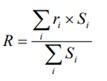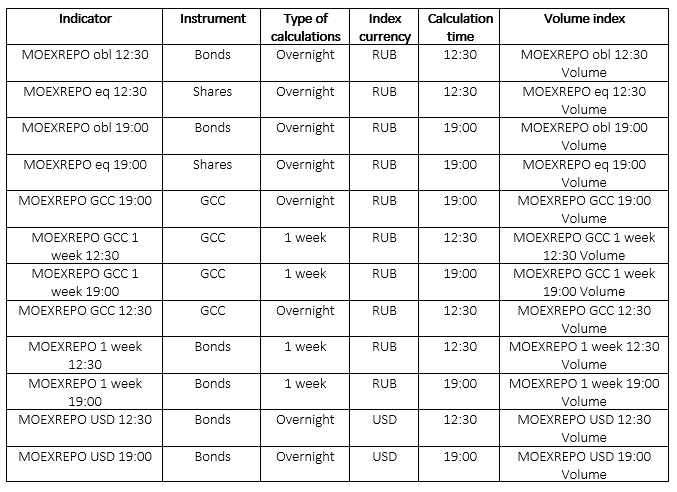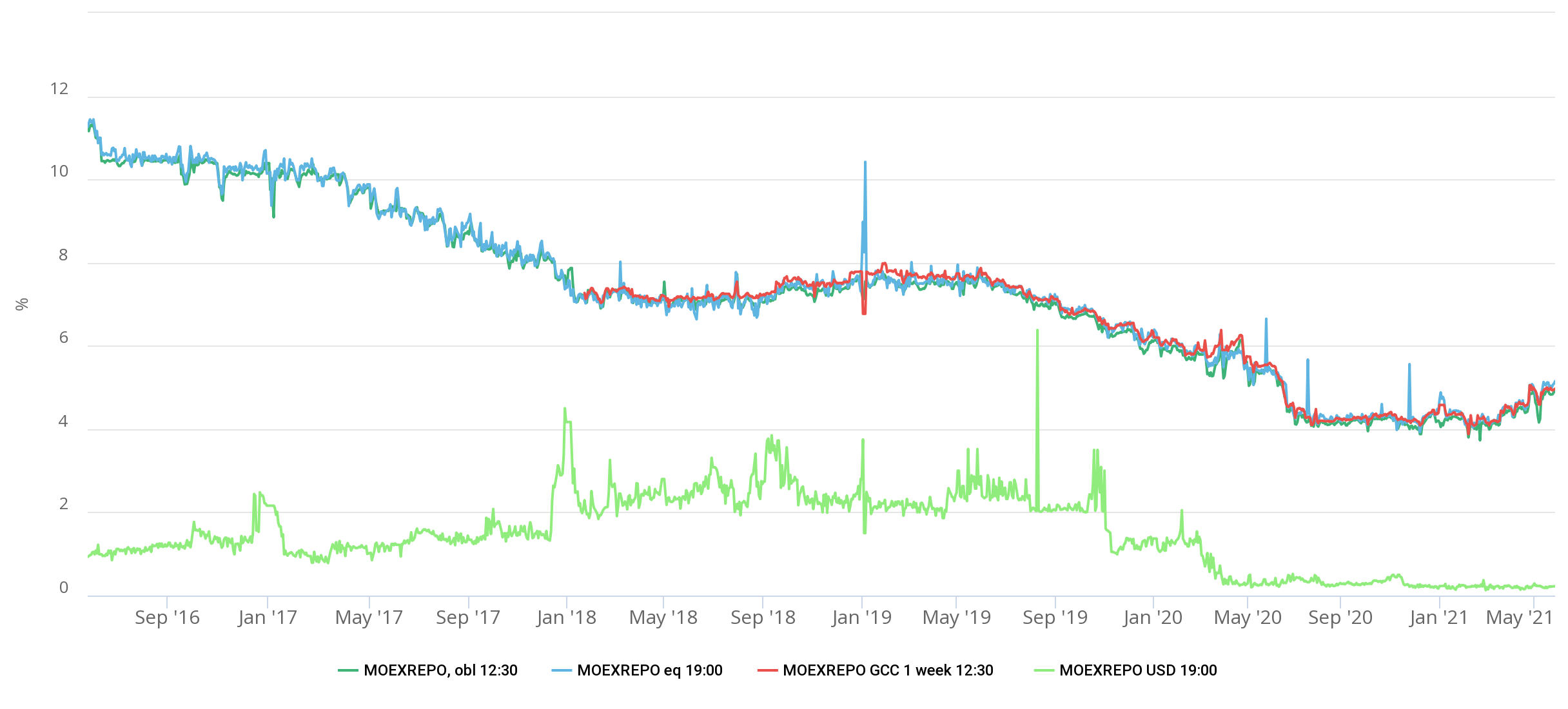Hint mode is switched on
Glossary

# MOEXREPO

Category — Rates
is an interest rate that is one of the indicators of the Russian money market. It acts as an indicator of the exchange market of repo with a central counterparty (CCP repo). This index is calculated by the Moscow Exchange every business day. The calculation of the rate is made as an annual percentage.

According to the Moscow Exchange methodology, the MOEXREPO indicator is calculated by the following formula:,

R is the value of the MOEXREPO index, ri is the repo with central counterparty rate, on the basis of which the indicator is calculated, and Si is the volume of transactions concluded at the ri rate.

MOEXREPO indicators are calculated for the following types of financial instruments:

1) bonds admitted to repo with a central counterparty, including OFZs and eurobonds;

2) shares admitted to repo with a central counterparty;

3) general collateral certificates (GCC)

There are 2 types of calculations of MOEXREPO indicators: overnight and 1 week. For the “overnight” calculation, the index is considered on the basis of repo operations in which the date of execution of the 2nd part of the transaction is the following compaction day after the date of the transaction. For the “1 week” settlement, the indicator is determined on the basis of repo transactions in which the date of execution of the 2nd part of the transaction protrude the 7th, 8th, and 9th day (the last two apply only to transactions with bonds and promotions) after the conclusion of the transaction.

MOEXREPO rates are calculated both for transactions in rubles and for operations in US dollars. At the same time, there are intraday MOEXREPO indicators, published at 12:30 (MSK), which take into account transactions executed on this day from 10:00 to 12:30, as well as end-of-day indicators, calculated at 19:00 (MSK), in which all transactions made in the period from 12:30 to 19:00 are included.

The Moscow Exchange also calculates and publishes the total volumes of transactions that are included in the calculation of MOEXREPO rates.

Taking into account all the above criteria, the following types of MOEXREPO indexes are distinguished:The graph below shows the dynamics of MOEXREPO index values for different types of tools and currencies.Registration is required to get access.